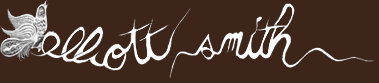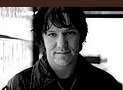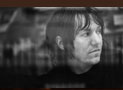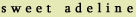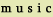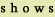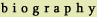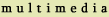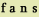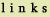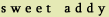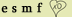v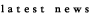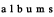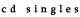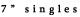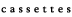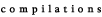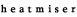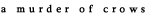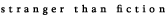figure 8 figure eight is double four figure four is half of eight If you skate you would be great if you could make a figure eight that's a circle that turns round upon itself figure eight is two times four four times four is two times eight If you skate upon thin ice you'd be wise if you thought twice before you made another single move thanks to daniel and steph